# Field (physics)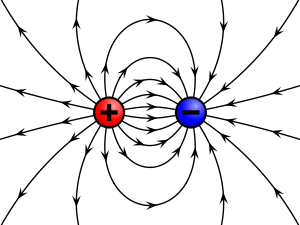Illustration of the electric field surrounding a positive (red) and a negative (blue) charge.

In physics, a field is a physical quantity, typically a number or tensor, that has a value for each point in space and time. For example, on a weather map, the surface wind velocity is described by assigning a vector to each point on a map. Each vector represents the speed and direction of the movement of air at that point. As another example, an electric field can be thought of as a "condition in space" emanating from an electric charge and extending throughout the whole of space. When a test electric charge is placed in this electric field, the particle accelerates due to a force. Physicists have found the notion of a field to be of such practical utility for the analysis of forces that they have come to think of a force as due to a field.

In the modern framework of the quantum theory of fields, even without referring to a test particle, a field occupies space, contains energy, and its presence eliminates a true vacuum. This led physicists to consider electromagnetic fields to be a physical entity, making the field concept a supporting paradigm of the edifice of modern physics. "The fact that the electromagnetic field can possess momentum and energy makes it very real ... a particle makes a field, and a field acts on another particle, and the field has such familiar properties as energy content and momentum, just as particles can have." In practice, the strength of most fields has been found to diminish with distance to the point of being undetectable. For instance the strength of many relevant classical fields, such as the gravitational field in Newton's theory of gravity or the electrostatic field in classical electromagnetism, is inversely proportional to the square of the distance from the source (i.e. they follow the Gauss's law). One consequence is that the Earth's gravitational field quickly becomes undetectable on cosmic scales.

A field can be classified as a scalar field, a vector field, a spinor field or a tensor field according to whether the represented physical quantity is a scalar, a vector, a spinor or a tensor, respectively. A field has a unique tensorial character in every point where it is defined: i.e. a field cannot be a scalar field somewhere and a vector field somewhere else. For example, the Newtonian gravitational field is a vector field: specifying its value at a point in spacetime requires three numbers, the components of the gravitational field vector at that point. Moreover, within each category (scalar, vector, tensor), a field can be either a classical field or a quantum field, depending on whether it is characterized by numbers or quantum operators respectively. In fact in this theory an equivalent representation of field is a field particle, namely a boson.

## History

To Isaac Newton his law of universal gravitation simply expressed the gravitational force that acted between any pair of massive objects. When looking at the motion of many bodies all interacting with each other, such as the planets in the Solar System, dealing with the force between each pair of bodies separately rapidly becomes computationally inconvenient. In the eighteenth century, a new quantity was devised to simplify the bookkeeping of all these gravitational forces. This quantity, the gravitational field, gave at each point in space the total gravitational force which would be felt by an object with unit mass at that point. This did not change the physics in any way: it did not matter if you calculated all the gravitational forces on an object individually and then added them together, or if you first added all the contributions together as a gravitational field and then applied it to an object.

The development of the independent concept of a field truly began in the nineteenth century with the development of the theory of electromagnetism. In the early stages, André-Marie Ampère and Charles-Augustin de Coulomb could manage with Newton-style laws that expressed the forces between pairs of electric charges or electric currents. However, it became much more natural to take the field approach and express these laws in terms of electric and magnetic fields; in 1849 Michael Faraday became the first to coin the term "field".

The independent nature of the field became more apparent with James Clerk Maxwell's discovery that waves in these fields propagated at a finite speed. Consequently, the forces on charges and currents no longer just depended on the positions and velocities of other charges and currents at the same time, but also on their positions and velocities in the past.

Maxwell, at first, did not adopt the modern concept of a field as fundamental quantity that could independently exist. Instead, he supposed that the electromagnetic field expressed the deformation of some underlying medium—the luminiferous aether—much like the tension in a rubber membrane. If that were the case, the observed velocity of the electromagnetic waves should depend upon the velocity of the observer with respect to the aether. Despite much effort, no experimental evidence of such an effect was ever found; the situation was resolved by the introduction of the special theory of relativity by Albert Einstein in 1905. This theory changed the way the viewpoints of moving observers should be related to each other in such a way that velocity of electromagnetic waves in Maxwell's theory would be the same for all observers. By doing away with the need for a background medium, this development opened the way for physicists to start thinking about fields as truly independent entities.

In the late 1920s, the new rules of quantum mechanics were first applied to the electromagnetic fields. In 1927, Paul Dirac used quantum fields to successfully explain how the decay of an atom to lower quantum state lead to the spontaneous emission of a photon, the quantum of the electromagnetic field. This was soon followed by the realization (following the work of Pascual Jordan, Eugene Wigner, Werner Heisenberg, and Wolfgang Pauli) that all particles, including electrons and protons, could be understood as the quanta of some quantum field, elevating fields to the status of the most fundamental objects in nature. That said, John Wheeler and Richard Feynman seriously considered Newton's pre-field concept of action at a distance (although they set it aside because of the ongoing utility of the field concept for research in general relativity and quantum electrodynamics).

## Classical fields

There are several examples of classical fields. Classical field theories remain useful wherever quantum properties do not arise, and can be active areas of research. Elasticity of materials, fluid dynamics and Maxwell's equations are cases in point.

Some of the simplest physical fields are vector force fields. Historically, the first time that fields were taken seriously was with Faraday's lines of force when describing the electric field. The gravitational field was then similarly described.

### Newtonian gravitation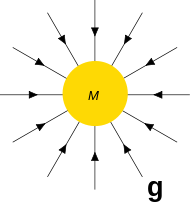In classical gravitation, mass is the source of an attractive gravitational field g.

A classical field theory describing gravity is Newtonian gravitation, which describes the gravitational force as a mutual interaction between two masses.

Any body with mass M is associated with a gravitational field g which describes its influence on other bodies with mass. The gravitational field of M at a point r in space corresponds to the ratio between force F that M exerts on a small or negligible test mass m located at r and the test mass itself:Stipulating that m is much smaller than M ensures that the presence of m has a negligible influence on the behavior of M.

According to Newton's law of universal gravitation, F(r) is given bywhereis a unit vector lying along the line joining M and m and pointing from m to M. Therefore, the gravitational field of M isThe experimental observation that inertial mass and gravitational mass are equal to an unprecedented level of accuracy leads to the identity that gravitational field strength is identical to the acceleration experienced by a particle. This is the starting point of the equivalence principle, which leads to general relativity.

Because the gravitational force F is conservative, the gravitational field g can be rewritten in terms of the gradient of a scalar function, the gravitational potential Φ(r):### Electromagnetism

Main article: Electromagnetism

Michael Faraday first realized the importance of a field as a physical quantity, during his investigations into magnetism. He realized that electric and magnetic fields are not only fields of force which dictate the motion of particles, but also have an independent physical reality because they carry energy.

These ideas eventually led to the creation, by James Clerk Maxwell, of the first unified field theory in physics with the introduction of equations for the electromagnetic field. The modern version of these equations is called Maxwell's equations.

#### Electrostatics

Main article: Electrostatics

A charged test particle with charge q experiences a force F based solely on its charge. We can similarly describe the electric field E so that F = qE. Using this and Coulomb's law tells us that the electric field due to a single charged particle asThe electric field is conservative, and hence can be described by a scalar potential, V(r):#### Magnetostatics

Main article: Magnetostatics

A steady current I flowing along a path will exert a force on nearby moving charged particles that is quantitatively different from the electric field force described above. The force exerted by I on a nearby charge q with velocity v iswhere B(r) is the magnetic field, which is determined from I by the Biot–Savart law:The magnetic field is not conservative in general, and hence cannot usually be written in terms of a scalar potential. However, it can be written in terms of a vector potential, A(r):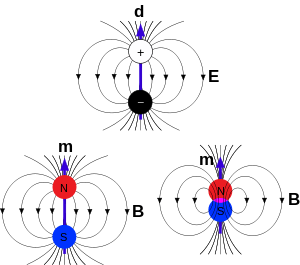The E fields and B fields due to electric charges (black/white) and magnetic poles (red/blue). Top: E field due to an electric dipole moment d. Bottom left: B field due to a mathematical magnetic dipole m formed by two magnetic monopoles. Bottom right: B field due to a pure magnetic dipole moment m found in ordinary matter (not from monopoles).

#### Electrodynamics

Main article: Electrodynamics

In general, in the presence of both a charge density ρ(r, t) and current density J(r, t), there will be both an electric and a magnetic field, and both will vary in time. They are determined by Maxwell's equations, a set of differential equations which directly relate E and B to ρ and J.

Alternatively, one can describe the system in terms of its scalar and vector potentials V and A. A set of integral equations known as retarded potentials allow one to calculate V and A from ρ and J,[note 1] and from there the electric and magnetic fields are determined via the relationsAt the end of the 19th century, the electromagnetic field was understood as a collection of two vector fields in space. Nowadays, one recognizes this as a single antisymmetric 2nd-rank tensor field in spacetime.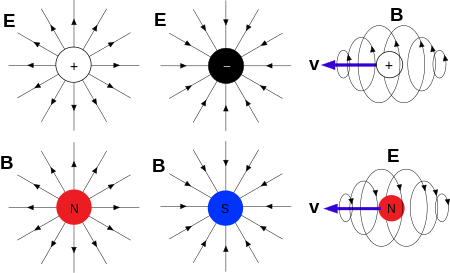The E fields and B fields due to electric charges (black/white) and magnetic poles (red/blue). E fields due to stationary electric charges and B fields due to stationary magnetic charges (note in nature N and S monopoles do not exist). In motion (velocity v), an electric charge induces a B field while a magnetic charge (not found in nature) would induce an E field. Conventional current is used.

### Gravitation in general relativity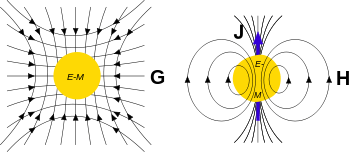In general relativity, mass-energy warps space time (Einstein tensor G), and rotating asymmetric mass-energy distributions with angular momentum J generate GEM fields H

Einstein's theory of gravity, called general relativity, is another example of a field theory. Here the principal field is the metric tensor, a symmetric 2nd-rank tensor field in spacetime. This replaces Newton's law of universal gravitation.

### Waves as fields

Waves can be constructed as physical fields, due to their finite propagation speed and causal nature when a simplified physical model of an isolated closed system is set . They are also subject to the inverse-square law.

For electromagnetic waves, there are optical fields, and terms such as near- and far-field limits for diffraction. In practice, though the field theories of optics are superseded by the electromagnetic field theory of Maxwell.

## Quantum fields

Main article: Quantum field theory

It is now believed that quantum mechanics should underlie all physical phenomena, so that a classical field theory should, at least in principle, permit a recasting in quantum mechanical terms; success yields the corresponding quantum field theory. For example, quantizing classical electrodynamics gives quantum electrodynamics. Quantum electrodynamics is arguably the most successful scientific theory; experimental data confirm its predictions to a higher precision (to more significant digits) than any other theory. The two other fundamental quantum field theories are quantum chromodynamics and the electroweak theory.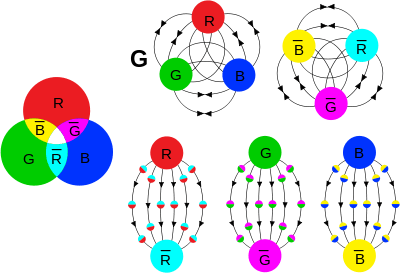Fields due to color charges, like in quarks (G is the gluon field strength tensor). These are "colorless" combinations. Top: Color charge has "ternary neutral states" as well as binary neutrality (analogous to electric charge). Bottom: The quark/antiquark combinations.

In quantum chromodynamics, the color field lines are coupled at short distances by gluons, which are polarized by the field and line up with it. This effect increases within a short distance (around 1 fm from the vicinity of the quarks) making the color force increase within a short distance, confining the quarks within hadrons. As the field lines are pulled together tightly by gluons, they do not "bow" outwards as much as an electric field between electric charges.

These three quantum field theories can all be derived as special cases of the so-called standard model of particle physics. General relativity, the Einsteinian field theory of gravity, has yet to be successfully quantized. However an extension, thermal field theory, deals with quantum field theory at finite temperatures, something seldom considered in quantum field theory.

In BRST theory one deals with odd fields, e.g. Faddeev–Popov ghosts. There are different descriptions of odd classical fields both on graded manifolds and supermanifolds.

As above with classical fields, it is possible to approach their quantum counterparts from a purely mathematical view using similar techniques as before. The equations governing the quantum fields are in fact PDEs (specifically, relativistic wave equations (RWEs)). Thus one can speak of Yang–Mills, Dirac, Klein–Gordon and Schrödinger fields as being solutions to their respective equations. A possible problem is that these RWEs can deal with complicated mathematical objects with exotic algebraic properties (e.g. spinors are not tensors, so may need calculus over spinor fields), but these in theory can still be subjected to analytical methods given appropriate mathematical generalization.

## Field theory

Field theory usually refers to a construction of the dynamics of a field, i.e. a specification of how a field changes with time or with respect to other independent physical variables on which the field depends. Usually this is done by writing a Lagrangian or a Hamiltonian of the field, and treating it as the classical mechanics (or quantum mechanics) of a system with an infinite number of degrees of freedom. The resulting field theories are referred to as classical or quantum field theories.

The dynamics of a classical field are usually specified by the Lagrangian density in terms of the field components; the dynamics can be obtained by using the action principle.

It is possible to construct simple fields without any a priori knowledge of physics using only mathematics from several variable calculus, potential theory and partial differential equations (PDEs). For example, scalar PDEs might consider quantities such as amplitude, density and pressure fields for the wave equation and fluid dynamics; temperature/concentration fields for the heat/diffusion equations. Outside of physics proper (e.g., radiometry and computer graphics), there are even light fields. All these previous examples are scalar fields. Similarly for vectors, there are vector PDEs for displacement, velocity and vorticity fields in (applied mathematical) fluid dynamics, but vector calculus may now be needed in addition, being calculus over vector fields (as are these three quantities, and those for vector PDEs in general). More generally problems in continuum mechanics may involve for example, directional elasticity (from which comes the term tensor, derived from the Latin word for stretch), complex fluid flows or anisotropic diffusion, which are framed as matrix-tensor PDEs, and then require matrices or tensor fields, hence matrix or tensor calculus. It should be noted that the scalars (and hence the vectors, matrices and tensors) can be real or complex as both are fields in the abstract-algebraic/ring-theoretic sense.

In a general setting, classical fields are described by sections of fiber bundles and their dynamics is formulated in the terms of jet manifolds (covariant classical field theory).

In modern physics, the most often studied fields are those that model the four fundamental forces which one day may lead to the Unified Field Theory.

### Symmetries of fields

A convenient way of classifying a field (classical or quantum) is by the symmetries it possesses. Physical symmetries are usually of two types:

#### Spacetime symmetries

Fields are often classified by their behaviour under transformations of spacetime. The terms used in this classification are:

• scalar fields (such as temperature) whose values are given by a single variable at each point of space. This value does not change under transformations of space.
• vector fields (such as the magnitude and direction of the force at each point in a magnetic field) which are specified by attaching a vector to each point of space. The components of this vector transform between themselves contravariantly under rotations in space. Similarly, a dual (or co-) vector field attaches a dual vector to each point of space, and the components of each dual vector transform covariantly.
• tensor fields, (such as the stress tensor of a crystal) specified by a tensor at each point of space. Under rotations in space, the components of the tensor transform in a more general way which depends on the number of covariant indices and contravariant indices.
• spinor fields (such as the Dirac spinor) arise in quantum field theory to describe particles with spin which transform like vectors except for the one of their component; in other words, when one rotates a vector field 360 degrees around a specific axis, the vector field turns to itself; however, spinors would turn to their negatives in the same case.

#### Internal symmetries

Main article: Internal symmetry

Fields may have internal symmetries in addition to spacetime symmetries. For example, in many situations one needs fields which are a list of space-time scalars: (φ1, φ2, ... φN). For example, in weather prediction these may be temperature, pressure, humidity, etc. In particle physics, the color symmetry of the interaction of quarks is an example of an internal symmetry of the strong interaction, as is the isospin or flavour symmetry.

If there is a symmetry of the problem, not involving spacetime, under which these components transform into each other, then this set of symmetries is called an internal symmetry. One may also make a classification of the charges of the fields under internal symmetries.

### Statistical field theory

Statistical field theory attempts to extend the field-theoretic paradigm toward many-body systems and statistical mechanics. As above, it can be approached by the usual infinite number of degrees of freedom argument.

Much like statistical mechanics has some overlap between quantum and classical mechanics, statistical field theory has links to both quantum and classical field theories, especially the former with which it shares many methods. One important example is mean field theory.

### Continuous random fields

Classical fields as above, such as the electromagnetic field, are usually infinitely differentiable functions, but they are in any case almost always twice differentiable. In contrast, generalized functions are not continuous. When dealing carefully with classical fields at finite temperature, the mathematical methods of continuous random fields are used, because thermally fluctuating classical fields are nowhere differentiable. Random fields are indexed sets of random variables; a continuous random field is a random field that has a set of functions as its index set. In particular, it is often mathematically convenient to take a continuous random field to have a Schwartz space of functions as its index set, in which case the continuous random field is a tempered distribution.

We can think about a continuous random field, in a (very) rough way, as an ordinary function that isalmost everywhere, but such that when we take a weighted average of all the infinities over any finite region, we get a finite result. The infinities are not well-defined; but the finite values can be associated with the functions used as the weight functions to get the finite values, and that can be well-defined. We can define a continuous random field well enough as a linear map from a space of functions into the real numbers.

## Notes

1. This is contingent on the correct choice of gauge. V and A are not completely determined by ρ and J; rather, they are only determined up to some scalar function f(r, t) known as the gauge. The retarded potential formalism requires one to choose the Lorenz gauge.

## References

1. John Gribbin (1998). Q is for Quantum: Particle Physics from A to Z. London: Weidenfeld & Nicolson. p. 138. ISBN 0-297-81752-3.
2. Richard Feynman (1970). The Feynman Lectures on Physics Vol II. Addison Wesley Longman. ISBN 978-0-201-02115-8. A “field” is any physical quantity which takes on different values at different points in space.
3. Ernan McMullin (2002). "The Origins of the Field Concept in Physics" (PDF). Phys. Perspect. 4: 13–39. Bibcode:2002PhP.....4...13M.
4. Richard P. Feynman (1970). The Feynman Lectures on Physics Vol II. Addison Wesley Longman.
5. Richard P. Feynman (1970). The Feynman Lectures on Physics Vol I. Addison Wesley Longman.
6. John Archibald Wheeler (1998). Geons, Black Holes, and Quantum Foam: A Life in Physics. London: Norton. p. 163.
7. Richard P. Feynman (1970). The Feynman Lectures on Physics Vol I. Addison Wesley Longman.
8. Steven Weinberg (November 7, 2013). "Physics: What We Do and Don't Know". New York Review of Books.
9. Weinberg, Steven (1977). "The Search for Unity: Notes for a History of Quantum Field Theory". Daedalus. 106 (4): 17–35. JSTOR 20024506.
10. Kleppner, David; Kolenkow, Robert. An Introduction to Mechanics. p. 85.
11. Parker, C.B. (1994). McGraw Hill Encyclopaedia of Physics (2nd ed.). Mc Graw Hill. ISBN 0-07-051400-3.
12. M. Mansfield; C. O’Sullivan (2011). Understanding Physics (4th ed.). John Wiley & Sons. ISBN 978-0-47-0746370.
13. Griffiths, David. Introduction to Electrodynamics (3rd ed.). p. 326.
14. Wangsness, Roald. Electromagnetic Fields (2nd ed.). p. 469.
15. J.A. Wheeler; C. Misner; K.S. Thorne (1973). Gravitation. W.H. Freeman & Co. ISBN 0-7167-0344-0.
16. I. Ciufolini; J.A. Wheeler (1995). Gravitation and Inertia. Princeton Physics Series. ISBN 0-691-03323-4.
17. Peskin, Michael E.; Schroeder, Daniel V. (1995). An Introduction to Quantum Fields. Westview Press. p. 198. ISBN 0-201-50397-2.. Also see precision tests of QED.
18. R. Resnick; R. Eisberg (1985). Quantum Physics of Atoms, Molecules, Solids, Nuclei and Particles (2nd ed.). John Wiley & Sons. p. 684. ISBN 978-0-471-87373-0.
19. Giachetta, G., Mangiarotti, L., Sardanashvily, G. (2009) Advanced Classical Field Theory. Singapore: World Scientific, ISBN 978-981-283-895-7 (arXiv: 0811.0331v2)